2022: SklogWiki celebrates 15 years on-line

# Partition function

The partition function of a system is given by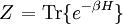$\left. Z \right.= {\mathrm {Tr}} \{ e^{-\beta H} \}$

where H is the Hamiltonian. The symbol Z is from the German Zustandssumme meaning "sum over states". The canonical ensemble partition function of a system in contact with a thermal bath at temperature$T$ is the normalization constant of the Boltzmann distribution function, and therefore its expression is given by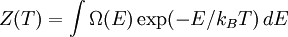$Z(T)=\int \Omega(E)\exp(-E/k_BT)\,dE$,

where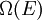$\Omega(E)$ is the density of states with energy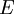$E$ and$k_B$ the Boltzmann constant.

In classical statistical mechanics, there is a close connection between the partition function and the configuration integral, which has played an important role in many applications (e.g., drug design).

## Helmholtz energy function

The partition function of a system is related to the Helmholtz energy function through the formula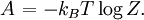$\left.A\right.=-k_BT\log Z.$

This connection can be derived from the fact that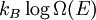$k_B\log\Omega(E)$ is the entropy of a system with total energy$E$. This is an extensive magnitude in the sense that, for large systems (i.e. in the thermodynamic limit, when the number of particles$N\to\infty$ or the volume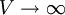$V\to\infty$), it is proportional to$N$ or$V$. In other words, if we assume$N$ large, then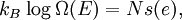$\left.k_B\right. \log\Omega(E)=Ns(e),$

where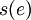$s(e)$ is the entropy per particle in the thermodynamic limit, which is a function of the energy per particle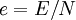$e=E/N$. We can therefore write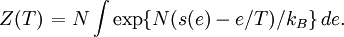$\left.Z(T)\right.=N\int \exp\{N(s(e)-e/T)/k_B\}\,de.$

Since$N$ is large, this integral can be performed through steepest descent, and we obtain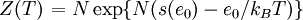$\left.Z(T)\right.=N\exp\{N(s(e_0)-e_0/k_BT)\}$,

where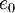$e_0$ is the value that maximizes the argument in the exponential; in other words, the solution to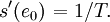$\left.s'(e_0)\right.=1/T.$

This is the thermodynamic formula for the inverse temperature provided$e_0$ is the mean energy per particle of the system. On the other hand, the argument in the exponential is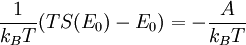$\frac{1}{k_BT}(TS(E_0)-E_0)=-\frac{A}{k_BT}$

the thermodynamic definition of the Helmholtz energy function. Thus, when$N$ is large,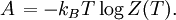$\left.A\right.=-k_BT\log Z(T).$

## Connection with thermodynamics

We have the aforementioned Helmholtz energy function,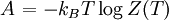$\left.A\right.=-k_BT\log Z(T)$

we also have the internal energy, which is given by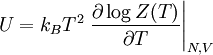$U=k_B T^{2} \left. \frac{\partial \log Z(T)}{\partial T} \right\vert_{N,V}$

and the pressure, which is given by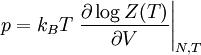$p=k_B T \left. \frac{\partial \log Z(T)}{\partial V} \right\vert_{N,T}$.

These equations provide a link between classical thermodynamics and statistical mechanics